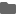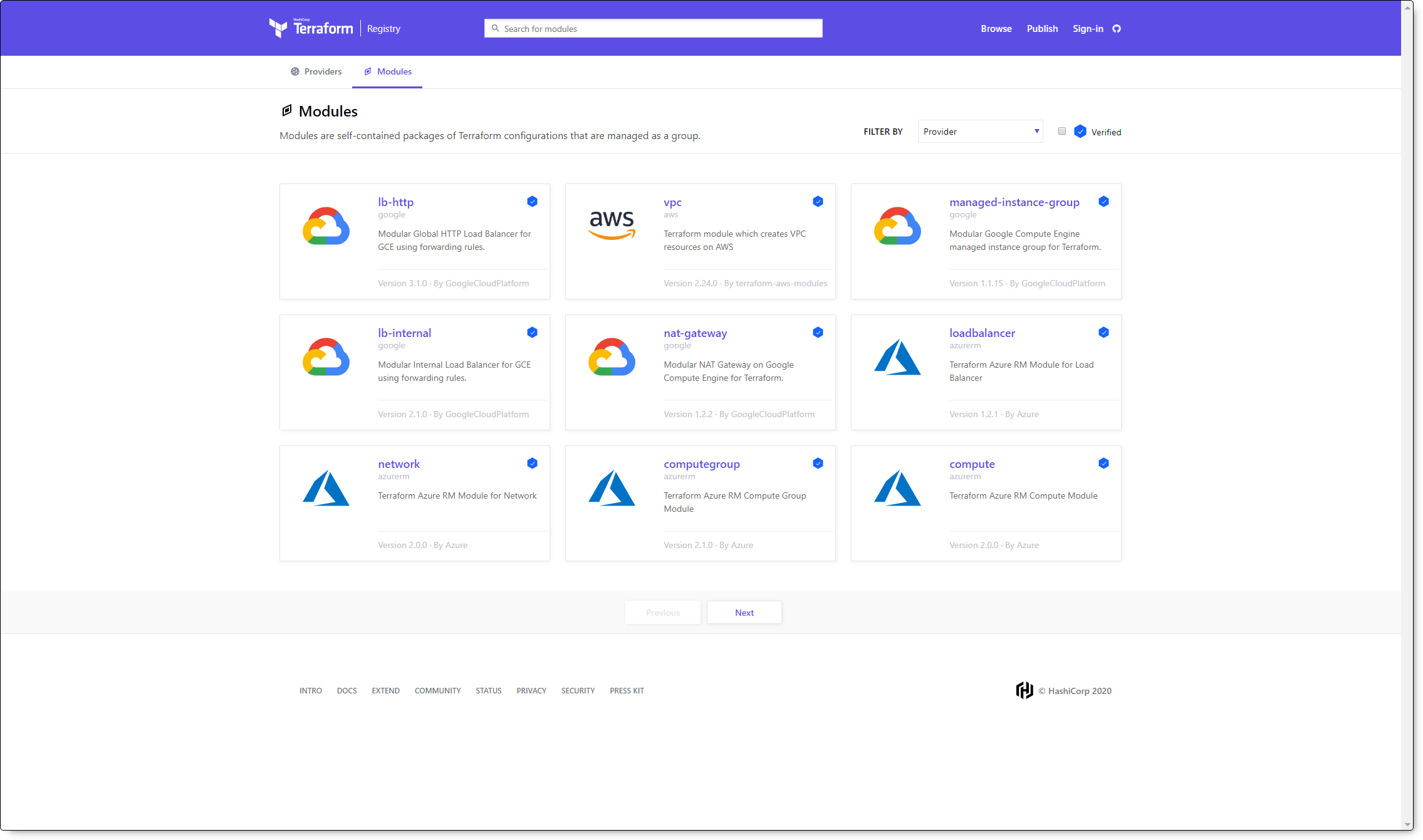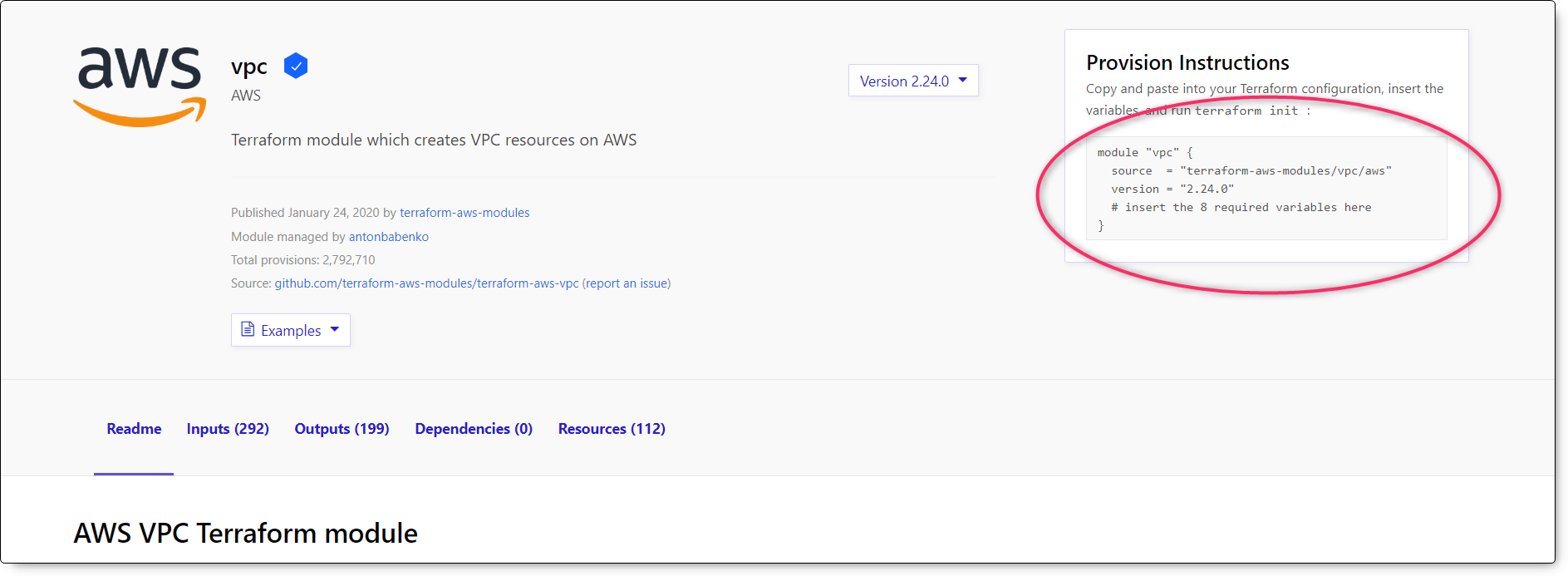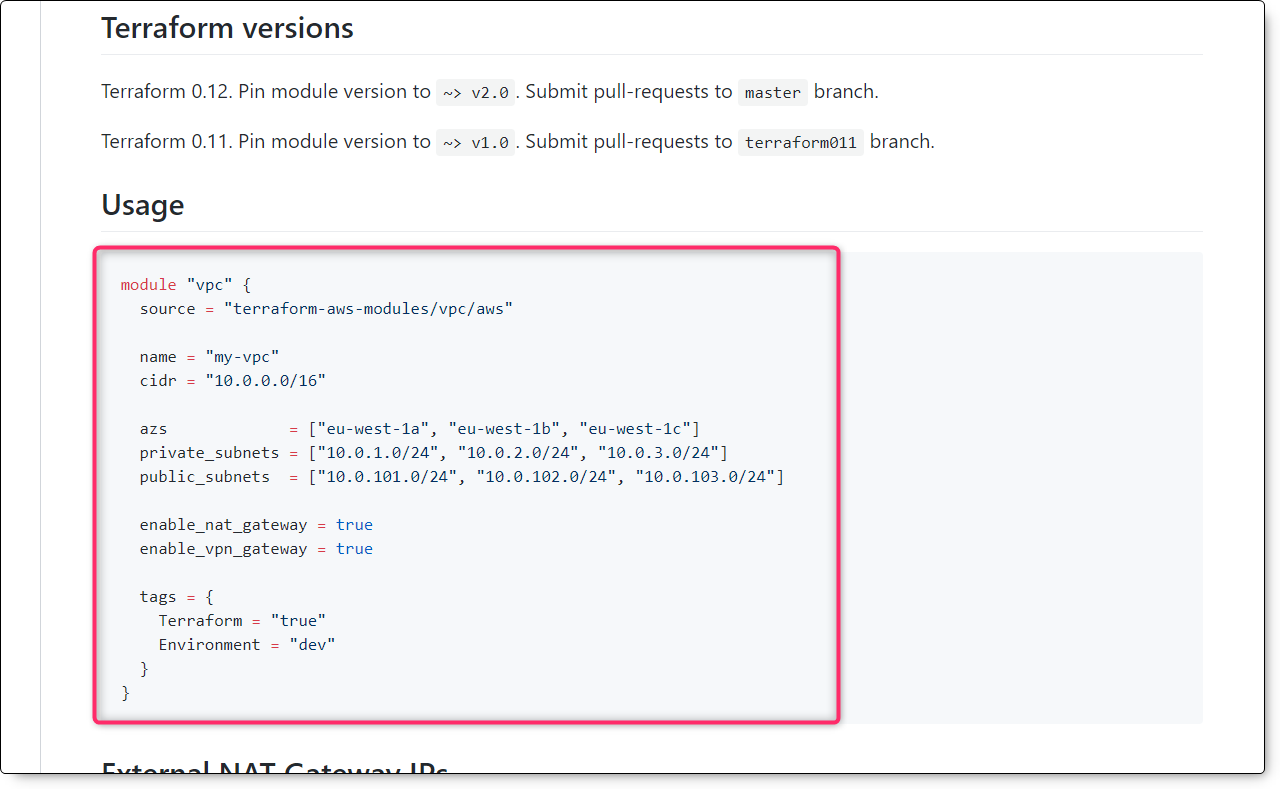# Terraform RegistryのModuleを使ってAWSリソースを作成してみた2020.02.06

## Terraform Moduleとは

Terraform RegistryのModuleの話に入る前にModuleについて簡単に説明します。

Terraform Moduleは関係するresourceのまとめを定義しておくテンプレートのようなものです。Moduleに必要な値を与えることで環境を構築することができます。さらにModuleは再利用できるので、環境の複製にとても便利だったり、パーツを組み合わせるようにModuleを使って様々なパターンの環境をさくっと作れたりします。環境の複製についてはWorkspaceもありますが、今回は説明を省略します。

とは言ってもModule本体はただの.tfファイルです。値を変数に置き換えておいて、Moduleを使う側で値を定義して使うイメージです。 文字ではイメージしにくいと思うので例を出します。※使い方には個人差があります。

### ディレクトリ構造

``````\$ tree
.
├── module.tf
└── modules
└── VPC
└── main.tf``````

### Moduleの中身

ディレクトリごとにModuleを分割しています。今回はVPCというディレクトリを作成することにしました。

`VPC/main.tf`

``````variable "vpc_cidr_block" {}
variable "vpc_name" {}

resource "aws_vpc" "this" {
cidr_block           = var.vpc_cidr_block
instance_tenancy     = "default"
enable_dns_hostnames = true

tags = {
Name = "\${var.vpc_name}-vpc"
}
}``````
• 空の変数を用意しておく
• Moduleを使う側で使用します。

なお今回は`enable_dns_hostnames = true`としていますが、ここをtrueではなく変数にしてより柔軟性を持たせるといったことも可能です。

### module.tfの中身(使う側)

`module.tf`

``````provider "aws" {
region     = var.aws_region
}
variable "aws_region" {
default = "ap-northeast-1"
}

## VPC
module "vpc_hoge" {
source         = "./modules/VPC"
vpc_cidr_block = "10.0.0.0/16"
vpc_name       = "hogehoge"
}``````
• sourceでModuleのあるディレクトリを指定
• Module側で定義している変数を渡してあげる

Moduleを呼び出してresourceを作成することができます。

※補足：ロールの認証で実行しているので、アクセスキー/シークレットキーは指定していません。

### 実行方法

• module.tfにあるディレクトリでコマンドを実行します。
• terraform initでModuleを呼び出します。
``````\$ terraform init
Initializing modules...
- vpc_hoge in modules/VPC

Initializing the backend...

Initializing provider plugins...

The following providers do not have any version constraints in configuration,

To prevent automatic upgrades to new major versions that may contain breaking
changes, it is recommended to add version = "..." constraints to the
corresponding provider blocks in configuration, with the constraint strings
suggested below.

* provider.aws: version = "~> 2.47"

Terraform has been successfully initialized!

You may now begin working with Terraform. Try running "terraform plan" to see
any changes that are required for your infrastructure. All Terraform commands
should now work.

If you ever set or change modules or backend configuration for Terraform,
rerun this command to reinitialize your working directory. If you forget, other
commands will detect it and remind you to do so if necessary.``````
• terraform planして内容を確認
``````\$ terraform plan
Refreshing Terraform state in-memory prior to plan...
The refreshed state will be used to calculate this plan, but will not be
persisted to local or remote state storage.

------------------------------------------------------------------------

An execution plan has been generated and is shown below.
Resource actions are indicated with the following symbols:
+ create

Terraform will perform the following actions:

# module.vpc_hoge.aws_vpc.this will be created
+ resource "aws_vpc" "this" {
+ arn                              = (known after apply)
+ assign_generated_ipv6_cidr_block = false
+ cidr_block                       = "10.0.0.0/16"
+ default_network_acl_id           = (known after apply)
+ default_route_table_id           = (known after apply)
+ default_security_group_id        = (known after apply)
+ dhcp_options_id                  = (known after apply)
+ enable_classiclink               = (known after apply)
+ enable_classiclink_dns_support   = (known after apply)
+ enable_dns_hostnames             = true
+ enable_dns_support               = true
+ id                               = (known after apply)
+ instance_tenancy                 = "default"
+ ipv6_association_id              = (known after apply)
+ ipv6_cidr_block                  = (known after apply)
+ main_route_table_id              = (known after apply)
+ owner_id                         = (known after apply)
+ tags                             = {
+ "Name" = "hogehoge_vpc-vpc"
}
}

Plan: 1 to add, 0 to change, 0 to destroy.

------------------------------------------------------------------------

Note: You didn't specify an "-out" parameter to save this plan, so Terraform
can't guarantee that exactly these actions will be performed if
"terraform apply" is subsequently run.``````

## Terraform RegistryのModule

どこかの誰かが作ったいい感じのModuleが使いたい...。そう思いませんか？それがTerraform Registryにあります！• 2020/02/06現在のModule
• AWS
• GCP
• Azure
• Alibaba
• ORACLE

また、HashiCorpによって検証されたModuleとユーザーコミュニティによって作成されたModuleがあります。検証されたModuleは使い方から詳細に書かれてあるので大体これで事が足ります。コミュニティによって作成されたModuleはちょっとニッチなサービスや特殊なケースで使われている構成等のものが公開されていたりします。

## Terraform RegistryのModuleを使ってみる

GitHubもあります。Moduleの中身を見たり、追加機能をプルリクしたりできます。terraform-aws-modules/terraform-aws-vpc

• VPC
• Subnet
• Route
• Route table
• Internet Gateway
• Network ACL
• NAT Gateway
• VPN Gateway
• VPC Endpoint
• ほぼ全て網羅されてるので省略
• RDS DB Subnet Group
• ElastiCache Subnet Group
• Redshift Subnet Group
• DHCP Options Set
• Default VPC
• Default Network ACL

ネットワークに関する設定大体書いてあるのではないでしょうか？

### 使い方

AWS VPC Terraform moduleの右上に簡単な手順が書いています。`module.tf`

``````module "vpc" {
source  = "terraform-aws-modules/vpc/aws"
version = "2.24.0"
# insert the 8 required variables here
}``````

もう少し下に詳細な使い方が記載されています。シンプルなものから詳細なものまで色々な構成を作成することができます。Module側の操作だけでここまで柔軟性を作るのは個人だと限界がありそうとModuleの中身を見る前に感じます...。

### Simple VPC

#### ディレクトリ構造

``````\$ tree
.
├── main.tf
└── output.tf``````

#### main.tf

`main.tf`

``````provider "aws" {
region = "eu-west-1"
}

data "aws_security_group" "default" {
name   = "default"
vpc_id = module.vpc.vpc_id
}

module "vpc" {
source = "terraform-aws-modules/vpc/aws"
name = "simple-example"
cidr = "10.0.0.0/16"

azs             = ["eu-west-1a", "eu-west-1b", "eu-west-1c"]
private_subnets = ["10.0.1.0/24", "10.0.2.0/24", "10.0.3.0/24"]
public_subnets  = ["10.0.101.0/24", "10.0.102.0/24", "10.0.103.0/24"]

enable_ipv6 = true

enable_nat_gateway = true
single_nat_gateway = true

public_subnet_tags = {
Name = "overridden-name-public"
}

tags = {
Owner       = "user"
Environment = "dev"
}

vpc_tags = {
Name = "vpc-name"
}
}``````
• Moduleのsource指定についてはディレクトリ指定ではなく手順に書いてあった通り直接指定してあります。`"terraform-aws-modules/vpc/aws"`

### 実行

• terraform initでModuleを呼び出します。
``````\$ terraform init
Initializing modules...
- vpc in .terraform/modules/vpc

Initializing the backend...

Initializing provider plugins...

Terraform has been successfully initialized!

You may now begin working with Terraform. Try running "terraform plan" to see
any changes that are required for your infrastructure. All Terraform commands
should now work.

If you ever set or change modules or backend configuration for Terraform,
rerun this command to reinitialize your working directory. If you forget, other
commands will detect it and remind you to do so if necessary.``````
• Moduleをダウンロードしているのが分かります。
• terraform planで作成されるリソースを確認します。
terraform plan(長いので折り畳み)
``````\$ terraform plan
Refreshing Terraform state in-memory prior to plan...
The refreshed state will be used to calculate this plan, but will not be
persisted to local or remote state storage.

------------------------------------------------------------------------

An execution plan has been generated and is shown below.
Resource actions are indicated with the following symbols:
+ create

Terraform will perform the following actions:

# data.aws_security_group.default will be read during apply
# (config refers to values not yet known)
<= data "aws_security_group" "default"  {
+ arn         = (known after apply)
+ description = (known after apply)
+ id          = (known after apply)
+ name        = "default"
+ tags        = (known after apply)
+ vpc_id      = (known after apply)
}

# module.vpc.aws_egress_only_internet_gateway.this will be created
+ resource "aws_egress_only_internet_gateway" "this" {
+ id     = (known after apply)
+ vpc_id = (known after apply)
}

# module.vpc.aws_eip.nat will be created
+ resource "aws_eip" "nat" {
+ allocation_id     = (known after apply)
+ association_id    = (known after apply)
+ domain            = (known after apply)
+ id                = (known after apply)
+ instance          = (known after apply)
+ network_interface = (known after apply)
+ private_dns       = (known after apply)
+ private_ip        = (known after apply)
+ public_dns        = (known after apply)
+ public_ip         = (known after apply)
+ public_ipv4_pool  = (known after apply)
+ tags              = {
+ "Environment" = "dev"
+ "Name"        = "simple-example-ap-northeast-1a"
+ "Owner"       = "user"
}
+ vpc               = true
}

# module.vpc.aws_internet_gateway.this will be created
+ resource "aws_internet_gateway" "this" {
+ id       = (known after apply)
+ owner_id = (known after apply)
+ tags     = {
+ "Environment" = "dev"
+ "Name"        = "simple-example"
+ "Owner"       = "user"
}
+ vpc_id   = (known after apply)
}

# module.vpc.aws_nat_gateway.this will be created
+ resource "aws_nat_gateway" "this" {
+ allocation_id        = (known after apply)
+ id                   = (known after apply)
+ network_interface_id = (known after apply)
+ private_ip           = (known after apply)
+ public_ip            = (known after apply)
+ subnet_id            = (known after apply)
+ tags                 = {
+ "Environment" = "dev"
+ "Name"        = "simple-example-ap-northeast-1a"
+ "Owner"       = "user"
}
}

# module.vpc.aws_route.private_ipv6_egress will be created
+ resource "aws_route" "private_ipv6_egress" {
+ destination_ipv6_cidr_block = "::/0"
+ destination_prefix_list_id  = (known after apply)
+ egress_only_gateway_id      = (known after apply)
+ gateway_id                  = (known after apply)
+ id                          = (known after apply)
+ instance_id                 = (known after apply)
+ instance_owner_id           = (known after apply)
+ nat_gateway_id              = (known after apply)
+ network_interface_id        = (known after apply)
+ origin                      = (known after apply)
+ route_table_id              = (known after apply)
+ state                       = (known after apply)
}

# module.vpc.aws_route.private_ipv6_egress will be created
+ resource "aws_route" "private_ipv6_egress" {
+ destination_ipv6_cidr_block = "::/0"
+ destination_prefix_list_id  = (known after apply)
+ egress_only_gateway_id      = (known after apply)
+ gateway_id                  = (known after apply)
+ id                          = (known after apply)
+ instance_id                 = (known after apply)
+ instance_owner_id           = (known after apply)
+ nat_gateway_id              = (known after apply)
+ network_interface_id        = (known after apply)
+ origin                      = (known after apply)
+ route_table_id              = (known after apply)
+ state                       = (known after apply)
}

# module.vpc.aws_route.private_ipv6_egress will be created
+ resource "aws_route" "private_ipv6_egress" {
+ destination_ipv6_cidr_block = "::/0"
+ destination_prefix_list_id  = (known after apply)
+ egress_only_gateway_id      = (known after apply)
+ gateway_id                  = (known after apply)
+ id                          = (known after apply)
+ instance_id                 = (known after apply)
+ instance_owner_id           = (known after apply)
+ nat_gateway_id              = (known after apply)
+ network_interface_id        = (known after apply)
+ origin                      = (known after apply)
+ route_table_id              = (known after apply)
+ state                       = (known after apply)
}

# module.vpc.aws_route.private_nat_gateway will be created
+ resource "aws_route" "private_nat_gateway" {
+ destination_cidr_block     = "0.0.0.0/0"
+ destination_prefix_list_id = (known after apply)
+ egress_only_gateway_id     = (known after apply)
+ gateway_id                 = (known after apply)
+ id                         = (known after apply)
+ instance_id                = (known after apply)
+ instance_owner_id          = (known after apply)
+ nat_gateway_id             = (known after apply)
+ network_interface_id       = (known after apply)
+ origin                     = (known after apply)
+ route_table_id             = (known after apply)
+ state                      = (known after apply)

+ timeouts {
+ create = "5m"
}
}

# module.vpc.aws_route.public_internet_gateway will be created
+ resource "aws_route" "public_internet_gateway" {
+ destination_cidr_block     = "0.0.0.0/0"
+ destination_prefix_list_id = (known after apply)
+ egress_only_gateway_id     = (known after apply)
+ gateway_id                 = (known after apply)
+ id                         = (known after apply)
+ instance_id                = (known after apply)
+ instance_owner_id          = (known after apply)
+ nat_gateway_id             = (known after apply)
+ network_interface_id       = (known after apply)
+ origin                     = (known after apply)
+ route_table_id             = (known after apply)
+ state                      = (known after apply)

+ timeouts {
+ create = "5m"
}
}

# module.vpc.aws_route.public_internet_gateway_ipv6 will be created
+ resource "aws_route" "public_internet_gateway_ipv6" {
+ destination_ipv6_cidr_block = "::/0"
+ destination_prefix_list_id  = (known after apply)
+ egress_only_gateway_id      = (known after apply)
+ gateway_id                  = (known after apply)
+ id                          = (known after apply)
+ instance_id                 = (known after apply)
+ instance_owner_id           = (known after apply)
+ nat_gateway_id              = (known after apply)
+ network_interface_id        = (known after apply)
+ origin                      = (known after apply)
+ route_table_id              = (known after apply)
+ state                       = (known after apply)
}

# module.vpc.aws_route_table.private will be created
+ resource "aws_route_table" "private" {
+ id               = (known after apply)
+ owner_id         = (known after apply)
+ propagating_vgws = (known after apply)
+ route            = (known after apply)
+ tags             = {
+ "Environment" = "dev"
+ "Name"        = "simple-example-private"
+ "Owner"       = "user"
}
+ vpc_id           = (known after apply)
}

# module.vpc.aws_route_table.public will be created
+ resource "aws_route_table" "public" {
+ id               = (known after apply)
+ owner_id         = (known after apply)
+ propagating_vgws = (known after apply)
+ route            = (known after apply)
+ tags             = {
+ "Environment" = "dev"
+ "Name"        = "simple-example-public"
+ "Owner"       = "user"
}
+ vpc_id           = (known after apply)
}

# module.vpc.aws_route_table_association.private will be created
+ resource "aws_route_table_association" "private" {
+ id             = (known after apply)
+ route_table_id = (known after apply)
+ subnet_id      = (known after apply)
}

# module.vpc.aws_route_table_association.private will be created
+ resource "aws_route_table_association" "private" {
+ id             = (known after apply)
+ route_table_id = (known after apply)
+ subnet_id      = (known after apply)
}

# module.vpc.aws_route_table_association.private will be created
+ resource "aws_route_table_association" "private" {
+ id             = (known after apply)
+ route_table_id = (known after apply)
+ subnet_id      = (known after apply)
}

# module.vpc.aws_route_table_association.public will be created
+ resource "aws_route_table_association" "public" {
+ id             = (known after apply)
+ route_table_id = (known after apply)
+ subnet_id      = (known after apply)
}

# module.vpc.aws_route_table_association.public will be created
+ resource "aws_route_table_association" "public" {
+ id             = (known after apply)
+ route_table_id = (known after apply)
+ subnet_id      = (known after apply)
}

# module.vpc.aws_route_table_association.public will be created
+ resource "aws_route_table_association" "public" {
+ id             = (known after apply)
+ route_table_id = (known after apply)
+ subnet_id      = (known after apply)
}

# module.vpc.aws_subnet.private will be created
+ resource "aws_subnet" "private" {
+ arn                             = (known after apply)
+ availability_zone               = "ap-northeast-1a"
+ availability_zone_id            = (known after apply)
+ cidr_block                      = "10.0.1.0/24"
+ id                              = (known after apply)
+ ipv6_cidr_block                 = (known after apply)
+ ipv6_cidr_block_association_id  = (known after apply)
+ map_public_ip_on_launch         = false
+ owner_id                        = (known after apply)
+ tags                            = {
+ "Environment" = "dev"
+ "Name"        = "simple-example-private-ap-northeast-1a"
+ "Owner"       = "user"
}
+ vpc_id                          = (known after apply)
}

# module.vpc.aws_subnet.private will be created
+ resource "aws_subnet" "private" {
+ arn                             = (known after apply)
+ availability_zone               = "ap-northeast1c"
+ availability_zone_id            = (known after apply)
+ cidr_block                      = "10.0.2.0/24"
+ id                              = (known after apply)
+ ipv6_cidr_block                 = (known after apply)
+ ipv6_cidr_block_association_id  = (known after apply)
+ map_public_ip_on_launch         = false
+ owner_id                        = (known after apply)
+ tags                            = {
+ "Environment" = "dev"
+ "Name"        = "simple-example-private-ap-northeast1c"
+ "Owner"       = "user"
}
+ vpc_id                          = (known after apply)
}

# module.vpc.aws_subnet.private will be created
+ resource "aws_subnet" "private" {
+ arn                             = (known after apply)
+ availability_zone               = "ap-northeast-1d"
+ availability_zone_id            = (known after apply)
+ cidr_block                      = "10.0.3.0/24"
+ id                              = (known after apply)
+ ipv6_cidr_block                 = (known after apply)
+ ipv6_cidr_block_association_id  = (known after apply)
+ map_public_ip_on_launch         = false
+ owner_id                        = (known after apply)
+ tags                            = {
+ "Environment" = "dev"
+ "Name"        = "simple-example-private-ap-northeast-1d"
+ "Owner"       = "user"
}
+ vpc_id                          = (known after apply)
}

# module.vpc.aws_subnet.public will be created
+ resource "aws_subnet" "public" {
+ arn                             = (known after apply)
+ availability_zone               = "ap-northeast-1a"
+ availability_zone_id            = (known after apply)
+ cidr_block                      = "10.0.101.0/24"
+ id                              = (known after apply)
+ ipv6_cidr_block                 = (known after apply)
+ ipv6_cidr_block_association_id  = (known after apply)
+ map_public_ip_on_launch         = true
+ owner_id                        = (known after apply)
+ tags                            = {
+ "Environment" = "dev"
+ "Name"        = "overridden-name-public"
+ "Owner"       = "user"
}
+ vpc_id                          = (known after apply)
}

# module.vpc.aws_subnet.public will be created
+ resource "aws_subnet" "public" {
+ arn                             = (known after apply)
+ availability_zone               = "ap-northeast1c"
+ availability_zone_id            = (known after apply)
+ cidr_block                      = "10.0.102.0/24"
+ id                              = (known after apply)
+ ipv6_cidr_block                 = (known after apply)
+ ipv6_cidr_block_association_id  = (known after apply)
+ map_public_ip_on_launch         = true
+ owner_id                        = (known after apply)
+ tags                            = {
+ "Environment" = "dev"
+ "Name"        = "overridden-name-public"
+ "Owner"       = "user"
}
+ vpc_id                          = (known after apply)
}

# module.vpc.aws_subnet.public will be created
+ resource "aws_subnet" "public" {
+ arn                             = (known after apply)
+ availability_zone               = "ap-northeast-1d"
+ availability_zone_id            = (known after apply)
+ cidr_block                      = "10.0.103.0/24"
+ id                              = (known after apply)
+ ipv6_cidr_block                 = (known after apply)
+ ipv6_cidr_block_association_id  = (known after apply)
+ map_public_ip_on_launch         = true
+ owner_id                        = (known after apply)
+ tags                            = {
+ "Environment" = "dev"
+ "Name"        = "overridden-name-public"
+ "Owner"       = "user"
}
+ vpc_id                          = (known after apply)
}

# module.vpc.aws_vpc.this will be created
+ resource "aws_vpc" "this" {
+ arn                              = (known after apply)
+ assign_generated_ipv6_cidr_block = true
+ cidr_block                       = "10.0.0.0/16"
+ default_network_acl_id           = (known after apply)
+ default_route_table_id           = (known after apply)
+ default_security_group_id        = (known after apply)
+ dhcp_options_id                  = (known after apply)
+ enable_classiclink               = (known after apply)
+ enable_classiclink_dns_support   = (known after apply)
+ enable_dns_hostnames             = false
+ enable_dns_support               = true
+ id                               = (known after apply)
+ instance_tenancy                 = "default"
+ ipv6_association_id              = (known after apply)
+ ipv6_cidr_block                  = (known after apply)
+ main_route_table_id              = (known after apply)
+ owner_id                         = (known after apply)
+ tags                             = {
+ "Environment" = "dev"
+ "Name"        = "vpc-name"
+ "Owner"       = "user"
}
}

Plan: 25 to add, 0 to change, 0 to destroy.

------------------------------------------------------------------------

Note: You didn't specify an "-out" parameter to save this plan, so Terraform
can't guarantee that exactly these actions will be performed if
"terraform apply" is subsequently run.``````

25個のリソースが作成されるようです。

• VPC
• サブネット
• private 3つ
• public 3つ
• Egress-Only インターネットゲートウェイ
• IPv6route/ルートテーブル/アタッチ
• インターネットゲートウェイ
• route/ルートテーブル/アタッチ
• IPv6route
• NATゲートウェイ
• route/ルートテーブル/アタッチ
• EIP
• NATゲートウェイにアタッチ

main.tfに記載した内容がそのまま反映されています。項目を埋めるだけでしたが、ルートテーブルのアタッチまでやってくれています。かなり便利。